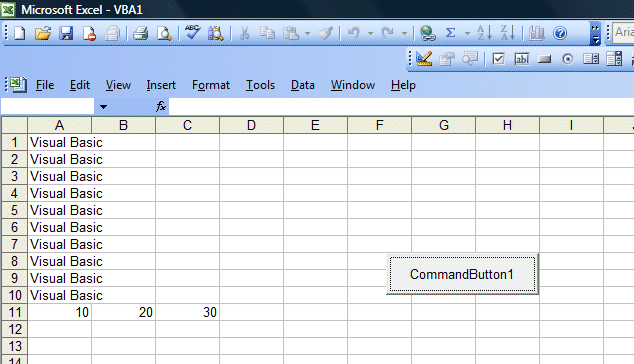Lesson 1: Introduction

Excel VBA means Excel Visual Basic for Applications which can be created using the built-in Visual Basic Editor in Microsoft Excel. An application created by Excel VBA is also known as macro. This tutorial is based on MS Excel 2003. If you wish to learn VBA for MS Excel 2010, follow our Excel VBA 2010 Tutorial here.

There are two ways which you can create an Excel VBA macro. The first is to place a command button on the MS Excel spreadsheet and click on the button to enter the Visual Basic Editor. The second is to launch the Visual Basic Editor from the menu. To launch the VBE, click on tools on the menu bar and select macro and then click on Visual Basic Editor.

1.1 Creating Excel VBA Using the Command Button

To create an Excel VBA using the command button, you need to place it on the spreadsheet. In order to access the command button, you need to click View on the MS Excel menu bar and then click on the toolbar and select the Control Toolbox to launch the control toolbox, as shown in Figure 1.1 .The control toolbox comprises various controls, as shown in Figure 1.2. Select the command button and place it on the spreadsheet.Figure 1.1>

Figure 1.2: The Control Toolbox

Next, you click on the command button and the Visual Basic Editor will appear.Enter the statement as shown in the Figure 1.3. The first statement will fill up cell A1 to cell A10 with the phrase "Visual Basic" while the second statement add the value in cell A11 and cell B11  and then show the sum in cell C11. The output is shown in Figure 1.4.Figure 1.3 Visual Basic Editor1.2 Creating Excel VBA in Visual Basic Editor

To launch the VBE, click on tools on the menu bar and select macro and then click on Visual Basic Editor,as shown in Figure 1.5. The Visual Basic Editor code window is shown in Figure 1.6. You can create a VBA function in the VBE. We will learn about function in later lesson.Figure 1.5